Home > CC2 > Chapter 7 > Lesson 7.1.3 > Problem7-35

7-35.
1. Study the following division problems and the diagrams that represent them. Answer the question below each diagram. Homework Help ✎

1. 8 ÷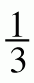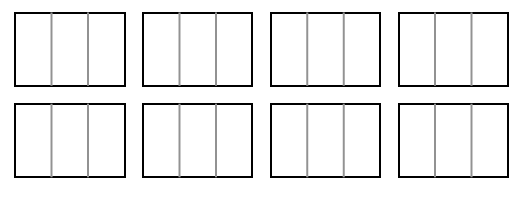How many thirds?

2. 8 ÷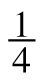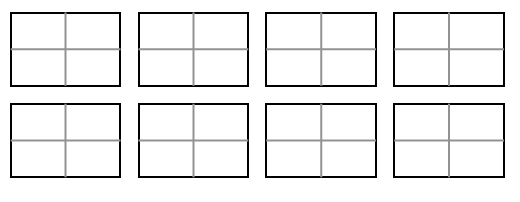How many fourths?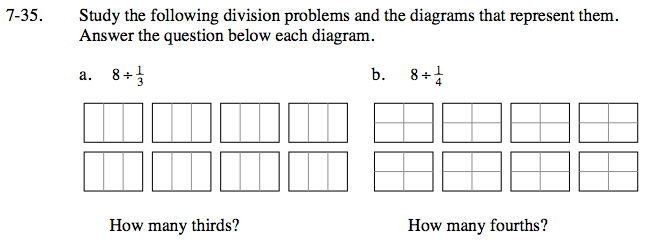8 wholes were each divided into 3 sections. Now count the number of sections to get your answer.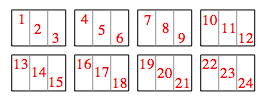There are 24 thirds.

See part (a).